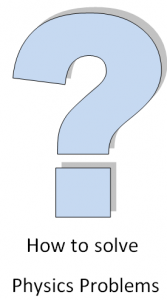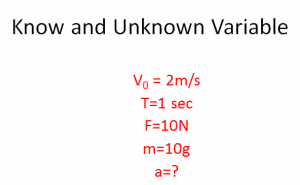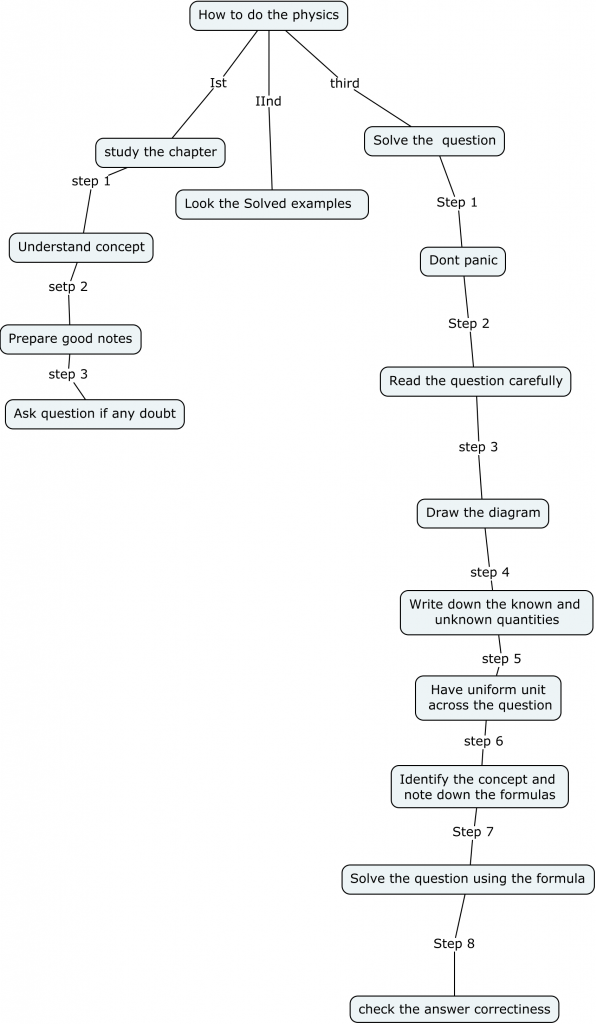Home » General » how to study physics problems

# how to study physics problemsFirst thing which student should do before doing the physics Problems is the study of the chapter and concepts

Here are the some of ways to study the chapter effectively

1) Attend all the classes in School. and understand whatever teacher is saying about the chapter
2) Take good notes. And develop the concept
3) When you copy drawings, completeness is worth more than careful artwork. You should not only copy what is on the board but also record important points that the teacher makes orally about the diagram
3) Study the chapter at home also and if you have any doubt ,ask the teacher and friends
4) Write down important formula and important concept in the chapter as it help in revision and solving the questions
5) Ask yourself honestly,if you truly understand the concept and ready to take on the problem . If it is still No,I would advise to take on the chapter again
Now that you have understood the chapter,Its time to pay attention to solved examples and get a way of solving the problems.Read the solved examples several times until it makes sense to you. Learn the way the solved examples is solved.After these two things are completed , we are ready to plunge into the problems.

Here are the steps I would advise to the student to excel in that
1) Don’t panic. Stay cool and have confidence that you will be able to solve the problems.
2) Read the problem carefully and understand what is being asked and tried to linked to the concept you have understood in the chapter. If don’t understand the problem first time,don’t panic read it again. Sometimes the wording of the problem may be tough but the question is simple. So you need to develop the attitude to carefully reading the problem and understand it quickly. It may take time initially but soon it will become the habit
3) Draw a clear diagram showing all the systems and interaction between them.For mechanics problem,Free body diagram provide immense help in solving the problem. Problem will become easier once it is drawn out. For electricity problem drawing the resistors in series and parallel will help. Drawing a clear diagram in the physics problem is very important as it help in understand the problem and makes the solving process easier. Many times drawing good diagram is even important for getting good marks

4) In any problem of physics, some information is given and value of some variable is to be found. Jot down all the known quantities on one side and List all the unknown for which you are solving the problem on the other side5) you must use consistent system for all the quantities involve. This will ensure you get correct answer
Suppose you have initial velocity is km/hr and final velocity in m/s ,then it makes sense to convert them into one unit
At the same time, if the physical quantities gives are in km/hr, then it is needed to convert them into m/s for the sake of conversion
6) Establish which general principle relates the given parameters to the quantity that you are seeking. Usually your picture will suggest the correct method and formulas. Write down relevant physics principles and identify physics equations that might be useful, particularly those that link the values given in the question with those that you are looking for. If the problem is around uniform motion in one dimension , we know that the below formula will be used

 v2=u2+2as s=ut+(1/2)at2 v=u+at

7) Take your formula and try to solve for one variable at a time. Solve for each variable that is listed under the “unknown” category. Try to solve for variables that you can determine easily first. We need to remember we should have as many equations as many unknown variables
Here are some of the tips for this
1) Do neat work so that things do not get messed in between
2) Distinguish between vector and scalar from starting and write proper notation and sign convention
3) Use proper variable and keep that till the end .It will help in doing the final calculation at the end
4) don’t use the same letter twice for different values in the same question.

8) Break down the large problem. Sometimes the problem may be big and it will look scary ,in these scenario, it is easy to divide that into pieces and then solve it linking each piece together

9) Finally check if the result makes sense to you
Following techniques can be used to check the correctness of the answer
Numerical reasonableness. If you need to find the mass of the person, then 1000 Kg will be unreasonable. if you’re working out a speed and reach a value greater than the speed of light, you know something has gone wrong

Dimensional analysis. Suppose you getup some answer in terms of some known physical quantities and constant. You should be able to perform dimensional analysis of the answer to check it correctness

The result obtained should hold good for the special cases and limiting values.So Does your answer give the expected values for extreme situations, such as when a quantity goes to zero or becomes very large?

Functionally reasonable: Many times the formula you receive as answer can be check using common sense.You may need to ask Does your answer depend on the given quantities in a reasonable way. Suppose in case of sound problem ,you know that if the object comes near you ,you will hear more frequency and if it is going far from
Always specify the result with units. If it is a vector quantity then please specify the direction also

10) Master these technique in physics homework ,then you should be able to finish your physics examination with in given time and with no mistakes
Here is the concept map on the above article on how to do physics problemsI have explained all the points in how to do physics problems above. Please do provide the feedback for this. Your feedback is very important for us

Do not miss this short presentation on how to memorize physics formulas
[iframe width=”560″ height=”315″ src=”https://www.youtube.com/embed/-Wqrw4G79Kc” frameborder=”0″ allowfullscreen][/iframe]

Subscribe
Notify of

This site uses Akismet to reduce spam. Learn how your comment data is processed.